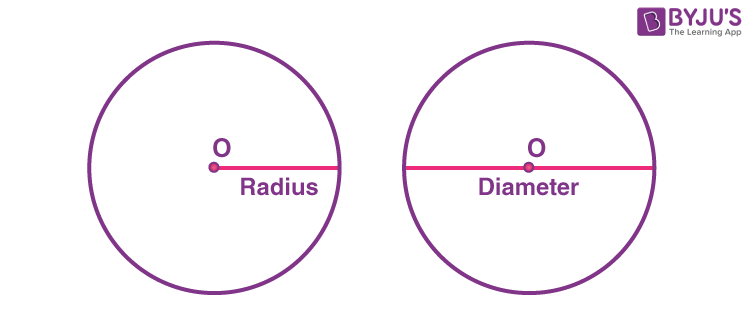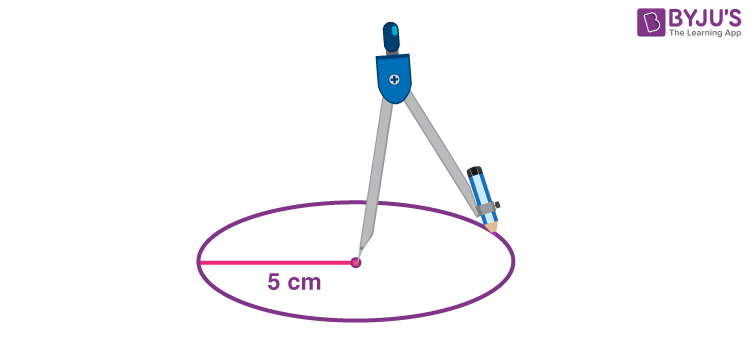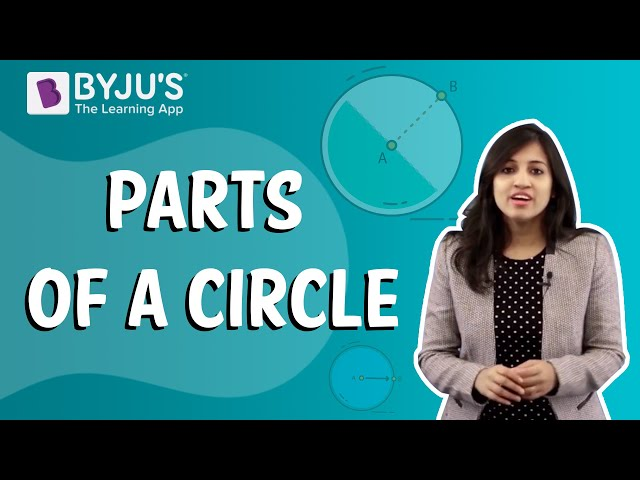# How To Draw A Perfect Circle?

Every day we come across different shapes in our day-to-day life. Many of us are already aware of a couple of them, such as Circles, Squares, triangles, parallelograms, hexagons, and so on. The characteristic property of different geometrical figures is length, breadth, diameter, etc., which make them unique from one another. Hence, to construct any of the geometrical figures, their distinctive properties must be known to us. Practical geometry is the most pragmatic branch of geometry that deals with constructing different geometrical figures using tools such as rulers, compasses and protractors. In this article, we will discuss how to draw a circle of a given radius.

The Circle

Before we move over to the procedure to learn how to draw a circle, it is required for you to keep some of its properties in mind.Radius and Diameter of a Circle

• The diameter is any straight line segment that passes through the center of the circle and joins two opposite points lying on the boundary of the circle.
•  The Radius of circle is a straight line segment that joins the center of the circle to any point on its boundary.
• Every point on the boundary of a circle is equidistant from its center.
• The length of any radius of a circle is half of its diameter.

## How to Draw a Circle of a Given Radius?

To draw a circle whose radius is given, we require a ruler and compasses. Given that the radius is 5 cm, the steps to be followed are:

• Step 1: Place the pointer of the compass at the initial point of the ruler (0 cm) and extend the other end of the pencil measuring 5 cm from the initial point (i.e. 5 cm)
• Step 2: Mark a point O on a piece of paper. This point is supposed to be the center of the circle that you are about to construct.
• Step 3: Place the pointer of the compass at point O.
• Step 4: Turn the compass slowly through 360 degrees to draw a circle.Make sure that you hold the point of the compass stiffly on the paper. You are also required to move the compass through the 360 degrees in one go for accuracy and neatness.

## Video Lessons on Circles

### Introduction to Circles### Parts of a Circle### Area of a CircleTo know more about methods to draw a circle and the circle formula, download BYJU’S-The Learning App.

## Frequently Asked Questions – FAQs

Q1

### What is the radius of a circle?

The radius of a circle is the distance from the center of the circle to any point on its circumference. It is usually denoted by ‘R’ or ‘r’.
Q2

### What is the diameter of a circle?

The diameter is the length of the line through the center that touches two points on the edge or circumference of the circle.
Q3

### What is the chord of a circle?

The chord of a circle can be defined as the line segment joining any two points on the circumference of the circle. Diameter is the longest chord of a circle which passes through the center of the circle
Q4

### What is the center of a circle?

The center of a circle is the point equidistant from the points on the edge or circumference.
Q5

### How many diameters and radii are there in the circle?

There can be an infinite number of lines joining them to the center. The diameter of the circle is twice as long as the radius. So, infinitely many diameters and radii can be formed in the circle.
Test your Knowledge on How to Draw a Perfect Circle